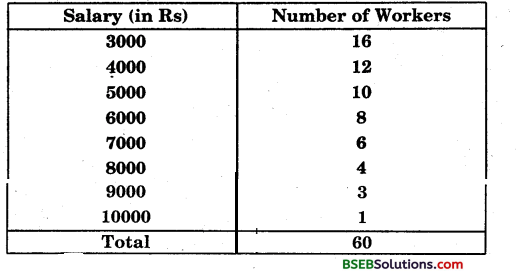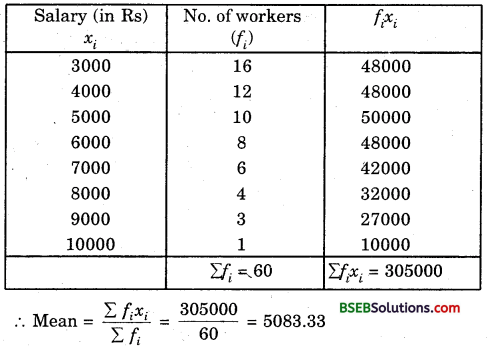# HSSlive: Plus One & Plus Two Notes & Solutions for Kerala State Board

## BSEB Class 9 Maths Chapter 14 Statistics Ex 14.4 Textbook Solutions PDF: Download Bihar Board STD 9th Maths Chapter 14 Statistics Ex 14.4 Book AnswersBSEB Class 9 Maths Chapter 14 Statistics Ex 14.4 Textbook Solutions PDF: Download Bihar Board STD 9th Maths Chapter 14 Statistics Ex 14.4 Book Answers

BSEB Class 9th Maths Chapter 14 Statistics Ex 14.4 Textbooks Solutions and answers for students are now available in pdf format. Bihar Board Class 9th Maths Chapter 14 Statistics Ex 14.4 Book answers and solutions are one of the most important study materials for any student. The Bihar Board Class 9th Maths Chapter 14 Statistics Ex 14.4 books are published by the Bihar Board Publishers. These Bihar Board Class 9th Maths Chapter 14 Statistics Ex 14.4 textbooks are prepared by a group of expert faculty members. Students can download these BSEB STD 9th Maths Chapter 14 Statistics Ex 14.4 book solutions pdf online from this page.

## Bihar Board Class 9th Maths Chapter 14 Statistics Ex 14.4 Books Solutions

 Board BSEB Materials Textbook Solutions/Guide Format DOC/PDF Class 9th Subject Maths Chapter 14 Statistics Ex 14.4 Chapters All Provider Hsslive

## How to download Bihar Board Class 9th Maths Chapter 14 Statistics Ex 14.4 Textbook Solutions Answers PDF Online?

2. Click on the Bihar Board Class 9th Maths Chapter 14 Statistics Ex 14.4 Answers.
3. Look for your Bihar Board STD 9th Maths Chapter 14 Statistics Ex 14.4 Textbooks PDF.
4. Now download or read the Bihar Board Class 9th Maths Chapter 14 Statistics Ex 14.4 Textbook Solutions for PDF Free.

Find below the list of all BSEB Class 9th Maths Chapter 14 Statistics Ex 14.4 Textbook Solutions for PDF’s for you to download and prepare for the upcoming exams:

## BSEB Bihar Board Class 9th Maths Solutions Chapter 14 Statistics Ex 14.4

Question 1.
The following number of goals were scored by a team in a series of 10 matches:
2, 3, 4, 5, 0, 1, 3, 3, 4, 3.
Find the mean, median and mode of these scores.
Solution:
Using 𝑥¯ = 𝑥1+𝑥2+…….+𝑥1010, the mean is
𝑥¯ = 2+3+4+5+04−1+3+3+4+310
= 2810 = 2.8
To find the median, arrange the given data in ascending order, as follows
0, 1, 2, 3, 3, 3, 3, 4, 4, 5.
There are 10 terms. So, there are two middle terms, i.e.,
the (102)th and (102 + 1)th
i.e., the 5th and 6th terms.
So, the median is the mean of the values of the 5th and 6th terms.
i.e., the median = 3+32 = 3
Again, in the data 3 occurs most frequently i.e., 4 times. So, mode = 3.

Question 2.
In a Mathematics test given to 15 students, the following marks (out of 100) are recorded:
41, 39, 48, 52, 46, 62, 54, 40, 96, 52, 98, 40, 42, 52, 60
Find the mean, median and mode of this data.
Solution:
Using 𝑥¯ = 𝑥1+𝑥2+…….+𝑥1510, the mean is
𝑥¯ = 41+39+48+52+46+62+54+40+96+52+98+40+42+52+6015
= 82215 = 54.8
To find the median, arrange the given data in ascending order, as follows:
39, 40, 40, 41, 42, 46, 48, 52, 52, 52, 54, 60, 62, 96, 98.
Since the number of terms is 15, an odd number, we find out the median by finding the marks obtained by (15+12)th student, which is 8th student.
∴ The median marks = 52
Again, in the data 52 occurs most frequently i.e., 3 times.
∴ Mode = 52.

Question 3.
The following observations have been arranged in ascending order. If the median of the data is 63, find the value of x.
29, 32, 48, 50, x, x + 2, 72, 78, 84, 95.
Solution:
The given arranged in ascending order is as
29, 32, 48, 50, x, x + 2, 72, 78, 84, 95
There are 10 terms. So, there are two middle terms, i.e.,
the (102)th and (102 + 1)th i.e., the 5th and 6th terms.
So, the median is the mean of the values of the 5th and 6 th terms.
i.e., the median = 𝑥+(𝑥+2)2 = x + 1
But median = 63
Given
∴ x + 1 = 63
⇒ x = 63 – 1 = 62.

Question 4.
Find the mode
14,25, 14, 28, 18, 17, 18, 14, 23, 22, 14, 18
Solution:
Arranging the given data in ascending order as follows, we get
14, 14, 14, 14, 17, 18, 18, 18, 22, 23, 25, 28.
Here 14 occurs most frequently i.e., 4 times.
∴ Mode = 14.

Question 5.
Find the mean salary of 60 workers of a factory from the following table:Solution:
Calculation of mean:Thus, mean salary of 60 workers is Rs 5083.33.

Question 6.
Give one example of a situation in which
(i) the mean is an appropriate measure of central tendency.
(ii) the mean is not an appropriate measure of central tendency but the median is an appropriate measure of central tendency.
Solution:
(i) The mean is an appropriate measure because of its unique value and can be used to compare different groups of data.
(ii) For the measurement of qualitative characteristics e.g., beauty, honesty, intelligence etc., mean cannot be used.

## Bihar Board Class 9th Maths Chapter 14 Statistics Ex 14.4 Textbooks for Exam Preparations

Bihar Board Class 9th Maths Chapter 14 Statistics Ex 14.4 Textbook Solutions can be of great help in your Bihar Board Class 9th Maths Chapter 14 Statistics Ex 14.4 exam preparation. The BSEB STD 9th Maths Chapter 14 Statistics Ex 14.4 Textbooks study material, used with the English medium textbooks, can help you complete the entire Class 9th Maths Chapter 14 Statistics Ex 14.4 Books State Board syllabus with maximum efficiency.

## FAQs Regarding Bihar Board Class 9th Maths Chapter 14 Statistics Ex 14.4 Textbook Solutions

#### Can we get a Bihar Board Book PDF for all Classes?

Yes you can get Bihar Board Text Book PDF for all classes using the links provided in the above article.

## Important Terms

Bihar Board Class 9th Maths Chapter 14 Statistics Ex 14.4, BSEB Class 9th Maths Chapter 14 Statistics Ex 14.4 Textbooks, Bihar Board Class 9th Maths Chapter 14 Statistics Ex 14.4, Bihar Board Class 9th Maths Chapter 14 Statistics Ex 14.4 Textbook solutions, BSEB Class 9th Maths Chapter 14 Statistics Ex 14.4 Textbooks Solutions, Bihar Board STD 9th Maths Chapter 14 Statistics Ex 14.4, BSEB STD 9th Maths Chapter 14 Statistics Ex 14.4 Textbooks, Bihar Board STD 9th Maths Chapter 14 Statistics Ex 14.4, Bihar Board STD 9th Maths Chapter 14 Statistics Ex 14.4 Textbook solutions, BSEB STD 9th Maths Chapter 14 Statistics Ex 14.4 Textbooks Solutions,
Share: